Search by Topic

Resources tagged with Perimeter similar to Area and Perimeter:

Filter by: Content type:
Age range:
Challenge level:

There are 24 results

Broad Topics > Measuring and calculating with units > Perimeter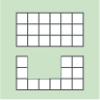Area and Perimeter

Age 7 to 11 Challenge Level:

What can you say about these shapes? This problem challenges you to create shapes with different areas and perimeters.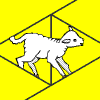Fencing Lambs

Age 7 to 11 Challenge Level:

A thoughtful shepherd used bales of straw to protect the area around his lambs. Explore how you can arrange the bales.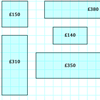Through the Window

Age 7 to 11 Challenge Level:

My local DIY shop calculates the price of its windows according to the area of glass and the length of frame used. Can you work out how they arrived at these prices?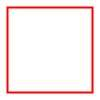Numerically Equal

Age 7 to 11 Challenge Level:

Can you draw a square in which the perimeter is numerically equal to the area?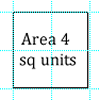Dicey Perimeter, Dicey Area

Age 7 to 11 Challenge Level:

In this game for two players, you throw two dice and find the product. How many shapes can you draw on the grid which have that area or perimeter?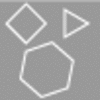Shapes on the Playground

Age 7 to 11 Challenge Level:

Sally and Ben were drawing shapes in chalk on the school playground. Can you work out what shapes each of them drew using the clues?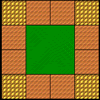Lawn Border

Age 5 to 11 Challenge Level:

If I use 12 green tiles to represent my lawn, how many different ways could I arrange them? How many border tiles would I need each time?Cutting it Out

Age 5 to 11 Challenge Level:

I cut this square into two different shapes. What can you say about the relationship between them?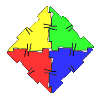Polydron

Age 7 to 11 Challenge Level:

This activity investigates how you might make squares and pentominoes from Polydron.Always, Sometimes or Never? Shape

Age 7 to 11 Challenge Level:

Are these statements always true, sometimes true or never true?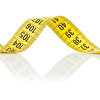Being Resilient - Primary Measures

Age 5 to 11 Challenge Level:

Measure problems at primary level that may require resilience.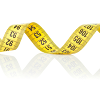Being Resourceful - Primary Measures

Age 5 to 11 Challenge Level:

Measure problems at primary level that require careful consideration.Can They Be Equal?

Age 11 to 14 Challenge Level:

Can you find rectangles where the value of the area is the same as the value of the perimeter?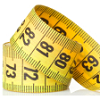Being Curious - Primary Measures

Age 5 to 11 Challenge Level:

Measure problems for inquiring primary learners.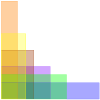Perimeter Possibilities

Age 11 to 14 Challenge Level:

I'm thinking of a rectangle with an area of 24. What could its perimeter be?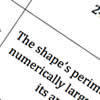Shape Draw

Age 7 to 11 Challenge Level:

Use the information on these cards to draw the shape that is being described.Is There a Theorem?

Age 11 to 14 Challenge Level:

Draw a square. A second square of the same size slides around the first always maintaining contact and keeping the same orientation. How far does the dot travel?Smaller and Smaller

Age 7 to 14 Challenge Level:

Can you predict, without drawing, what the perimeter of the next shape in this pattern will be if we continue drawing them in the same way?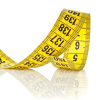Being Collaborative - Primary Measures

Age 5 to 11 Challenge Level:

Measure problems for primary learners to work on with others.On the Edge

Age 11 to 14 Challenge Level:

If you move the tiles around, can you make squares with different coloured edges?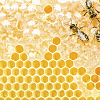Perimeter Challenge

Age 11 to 14 Challenge Level:

Can you deduce the perimeters of the shapes from the information given?Coins on a Plate

Age 11 to 14 Challenge Level:

Points A, B and C are the centres of three circles, each one of which touches the other two. Prove that the perimeter of the triangle ABC is equal to the diameter of the largest circle.Perimeter Expressions

Age 11 to 14 Challenge Level:

Create some shapes by combining two or more rectangles. What can you say about the areas and perimeters of the shapes you can make?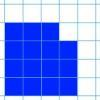Changing Areas, Changing Perimeters

Age 11 to 14 Challenge Level:

How can you change the area of a shape but keep its perimeter the same? How can you change the perimeter but keep the area the same?